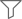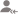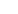trueAll

Lessons

DiscussionLesson Posted on 06/09/2018 ProgressionsRaj Kumar

I am Six Sigma Black belt trained from American Society of Quality I am 2011 pass out in B.tech from...

If a is the first term and r is the common ratio of the geometric progression, then its nth term is given by an = arn-1 The sum Sn of the first n terms of the G.P. is given by Sn = a (rn – 1)/ (r-1), when r ≠1; = na if r =1 If -1 < x < 1, then limxn = 0, as n →∞.... read more

If a is the first term and r  is the common ratio of the geometric progression,

then its nth term is given by an = arn-1

The sum Sn of the first n terms of the G.P. is given by

Sn = a (rn – 1)/ (r-1), when r ≠1; = na  if r =1

If -1 < x < 1, then limxn = 0, as n →∞. Hence, the sum of an infinite G.P. is 1+x+x2+ ….. = 1/(1-x)

If -1 < r< 1, then the sum of the infinite G.P. is a +ar+ ar2+ ….. = a/(1-r)

If each term of the G.P is multiplied or divided by a non-zero fixed constant, the resulting sequence is again a G.P.

If a1, a2, a3, …. andb1, b2, b3, … are two geometric progressions, then a1b1, a2b2, a3b3, …… is also a geometric progression and a1/b1, a2/b2, ... ... ..., an/bn will also be in G.P.

Suppose a1, a2, a3, ……,an are in G.P. then an, an–1, an–2, ……, a3, a2, a1 will also be in G.P.

Taking the inverse of a G.P. also results a G.P. Suppose a1, a2, a3, ……,an are in G.P then 1/a1, 1/a2, 1/a3 ……, 1/an will also be in G.P

If we need to assume three numbers in G.P. then they should be assumed as   a/b, a, ab  (here common ratio is b)

Four numbers in G.P. should be assumed as a/b3, a/b, ab, ab3 (here common ratio is b2)

Five numbers in G.P. a/b2, a/b, a, ab, ab2  (here common ratio is b)

If a1, a2, a3,… ,an is a G.P (ai> 0 ∀i), then log a1, log a2, log a3, ……, log an is an A.P. In this case, the converse of the statement also holds good.

If three terms are in G.P., then the middle term is called the geometric mean (G.M.) between the two. So if a, b, c are in G.P., then b

= √ac is the geometric mean of a and c.

Dislike Bookmark Share

Lesson Posted on 23/08/2018 ProgressionsRaj Kumar

I am Six Sigma Black belt trained from American Society of Quality I am 2011 pass out in B.tech from...

Let a, b and c form an H.P. Then 1/a, 1/b and 1/c form an A.P. If a, b and c are in H.P. then 2/b = 1/a + 1/c, which can be simplified as b = 2ac/(a+c) If a and b are two non-zero numbers then the sequence a, H, b is a H.P. The n numbers H1, H2, ……,Hn are said to... read more
• Let a, b and c form an H.P. Then 1/a, 1/b and 1/c form an A.P.

• If a, b and c are in H.P. then 2/b = 1/a + 1/c,  which can be simplified as b = 2ac/(a+c)

• If a  and b are two non-zero numbers then the sequence a, H, b is a H.P.

•     The n numbers H1, H2, ……,Hn are said to be harmonic means between a and b,
• if a, H1, H2 ……, Hn, b are in H.P. i.e. if 1/a, 1/H1, 1/H2, ..., 1/Hn, 1/b are in A.P.
• Let d be the common difference of the A.P., Then 1/b = 1/a + (n+1) d ⇒ d = a–b/(n+1)ab.
• If a  andb  are two positive real numbers then A.M x H.M = G.M2

• The relation between the three means is defined as A.M  >  G.M  >  H.M

• If we need to find three numbers in a H.P. then they should be assumed as 1/a–d, 1/a, 1/a+d

• Four convenient numbers in H.P. are 1/a–3d, 1/a–d, 1/a+d, 1/a+3d

• Five convenient numbers in H.P. are 1/a–2d, 1/a–d, 1/a, 1/a+d, 1/a+2d

Dislike Bookmark Share

Lesson Posted on 30/05/2018 ProgressionsHemant P.

1. I have taught at various prestigious institutes of repute in India and abroad. 2. Worked at (i)...

Geometric Mean (GM) The geometric mean is similar to the arithmetic mean, but it uses the property of multiplication, to find the mean between any two numbers. GM of two numbers a and b may be defined as G = √(ab)For example, GM of 2 and 3 is √6.We can find a general formula for inserting... read more

Geometric Mean (GM)

The geometric mean is similar to the arithmetic mean, but it uses the property of multiplication, to find the mean between any two numbers.

GM of two numbers a and b may be defined as G = √(ab)

For example, GM of 2 and 3 is √6.

We can find a general formula for inserting a GM between any two arbitrary numbers a and b.

If G is the geometric mean between a and b, then by definition of GP a,  G,  b are in GP.

Or G2=a.b

We can also find a formula for inserting n GM in between two numbers a and b.

Let  G1,  G2,  G3  ….  Gn be then GMs between a and b.

Now a,   G1,  G2, G3…. Gn,  b are in GP.

By definition of the last term of a GP  b = (n+2)th term of GP.

If R=common ratio, then by definition of GP,  b = a.Rn+1

Now G1=a?R

G2=a?R2

G3=a?R3

and Gn=a?Rn

We will take a few examples of GM too.

Find the GM of the following and insert n geometric means between them.

e and 1
7 and tan x
log x and sec-1x

Solution:

(i) The GM between e and 1 is e. To insert n GMs, we use the definition of geometric progressions.

Let  G1,   G2,  G3,  …  Gn,  be n  geometric means inserted between e and 1.

Also, let common ratio be R.

By the definition of GP    = (n+2)th term of the GP.

1 =eRn+1

Or R= (1/e)1/(n+1)

Hence   G1 = e⋅R = e⋅(1/e)1/(n+1)

G2=e⋅R2=e⋅(1/e)2/(n+1)

G3=e⋅R=e⋅(1/e)3/(n+1)

and  Gn=e⋅R=e⋅(1/e)n/(n+1)

(ii)   Proceeding as before the GM  of 7 and tanx is √(7tanx).

Let G1, G2, G3,… Gn,  be n  geometric means inserted between 7 and tanx.Also, let common ratio be R.

To get the n geometric means, we proceed as before.

Here tanx=(n+2)th term.

tanx=7⋅Rn+1

Or Rn+1= tanx/7

R = (tanx/7)1/n+1

G1 = 7R = 7⋅(tanx/7)1/n+1

G2  =  7R2   = 7⋅(tanx/7)2/n+1

G3 = 7R3 = 7⋅(tanx/7)3/n+1

and   Gn=7Rn=7(tanx/7)n/n+1

(iii)  Proceeding exactly as before, the GM of logx and sec-1x will be √(logx⋅sec-1x)

Let   G1,  G2,  G3, …  Gn,  be n  geometric means inserted between logx and sec-1x.

Also, let common ratio be R.

To insert n GMs between logx and sec-1x, we take the sequence as

logx, G1, G2, G3,… Gn, sec-1x.

Gn = (n+2)th term =  sec-1x = logx.Rn+1

R= (sec-1x/logx)1/(n+1)

Now as before G1=logx⋅R=logx⋅(sec-1x/logx)1/(n+1)

G2=logx⋅R2=logx⋅(sec-1x/logx)2/(n+1)

G3=logx⋅R3=logx⋅(sec-1x/logx)3/(n+1)

and Gn=logx⋅Rn=logx⋅(sec-1x/logx)n/(n+1)

Dislike Bookmark Share

Overview

Questions 30

Total Shares51 FollowersTapas Bhattacharya

Tutor

The first term, t1=a = 7. The common difference, b=13 - 7=6. Last term or the nth term, tn=a + (n-1)b = 205. So, we have, 7 + (n - 1)6 = 205 --> (n - 1) = (205 - 7)/6 = 33 --> n = 34. Answer: Number of terms is 34.
Dislike Bookmark Share

Find the A.P whose 10th term is 5 and 18th term is 77?Tapas Bhattacharya

Tutor

Let the first term be a and the common difference be b. So, the 10th term: t10 = a + 9b = 5. and, the 18th term: t18 = a + 17b = 77. So, from t17 - t10 we get: 8b = 77 - 5 = 72. This gives: b = 9. From t10 we get: a = 5 - 9b = 5 - 9x9 = -76. So, a = -76, b = 9 and the A.P is:- -76, -67, -58, -49,... read more
Let the first term be a and the common difference be b. So, the 10th term: t10 = a + 9b = 5. and, the 18th term: t18 = a + 17b = 77. So, from t17 - t10 we get: 8b = 77 - 5 = 72. This gives: b = 9. From t10 we get: a = 5 - 9b = 5 - 9x9 = -76. So, a = -76, b = 9 and the A.P is:- -76, -67, -58, -49, ..... (Answer). read less
Dislike Bookmark Share

In a certain A.P the 24th term is twice the 10th term. Prove that the 72nd term is twice the 34th term?Tapas Bhattacharya

Tutor

Let the first term be a and the common difference be b. So, by the given condition:- t24=2 x t10 --> a + 23 b = 2 ( a + 9b) = 2a + 18b. This gives: a = 5b. So, the 72nd term: t72 = a +71b = 5b + 71b = 76b. and the 34th term: t34 = a + 33b = 5b + 33b = 38b = 76b/2 = t72/2. So, the 72nd term is twice... read more
Let the first term be a and the common difference be b. So, by the given condition:- t24=2 x t10 --> a + 23 b = 2 ( a + 9b) = 2a + 18b. This gives: a = 5b. So, the 72nd term: t72 = a +71b = 5b + 71b = 76b. and the 34th term: t34 = a + 33b = 5b + 33b = 38b = 76b/2 = t72/2. So, the 72nd term is twice the 34th term. (Proved) read less
Dislike Bookmark Share

## Top Contributors

Connect with Expert Tutors & Institutes for ProgressionsThree numbers are in A.P. If the sum of these numbers be 27 and the product 648, find the numbers?Tapas Bhattacharya

Tutor

Let the 3 terms are (a - b), a, and (a + b). So, (a - b) + a + ( a + b) = 27=> 3a=27 => a=9. Now the product is 648. So, (9 - b) x 9 x (9 + b) = 648=> (81 - b^2) = 648/9 = 72=> b^2 = 81 - 72=9 => b = =3 or b = -3. For, b = 3, the numbers are: (9 - 3), 9, (9 + 3) = 6, 9, 12 or (9 - (-3)), 9... read more
Let the 3 terms are (a - b), a, and (a + b). So, (a - b) + a + ( a + b) = 27=> 3a=27 => a=9. Now the product is 648. So, (9 - b) x 9 x (9 + b) = 648=> (81 - b^2) = 648/9 = 72=> b^2 = 81 - 72=9 => b = =3 or b = -3. For, b = 3, the numbers are: (9 - 3), 9, (9 + 3) = 6, 9, 12 or (9 - (-3)), 9 , (9 + (-3)) = 12, 9, 6 The three numbers are 6, 9, 12 or 12, 9, 6 (Answer) read less
Dislike Bookmark Share

Write the first four terms of the A.p, when the first term a and the common difference d are given: a = 10, d = 10?Tapas Bhattacharya

Tutor

The first four terms are 10, 10 + 10, 10 + 2x10, and 10 + 3x10. So, the terms are: 10, 20, 30, 40.
Dislike Bookmark Share

For the given A.P write the first term and the common difference: 3, 1, -1, -3, …..?Tapas Bhattacharya

Tutor

The first term is 3. The common difference = 1-3 = -2.
Dislike Bookmark Share

If the 3rd and the 9th terms of an A.P are 4 and -8 respectively, which term of this A.P is zero?Tapas Bhattacharya

Tutor

Let the first term and the common difference are a and b. So, the third term: t3=a + 2b=4. and, the 9th term: t9=a + 8b=-8. So, t9 - t6=6b = -8 - 4=-12 --> b = - 2. So, from the expression of t3, we get: a = 4 - 2b = 4 + 4 = 8. Let us consider that the nth term is zero. So: tn... read more
Let the first term and the common difference are a and b. So, the third term: t3=a + 2b=4. and, the 9th term: t9=a + 8b=-8. So, t9 - t6=6b = -8 - 4=-12 --> b=- 2. So, from the expression of t3, we get: a=4 - 2b=4 + 4=8. Let us consider that the nth term is zero. So: tn=a + (n-1)b = 0 --> 8 + (n-1)(-2) = 0 --> 8 -2n +2 = 0 --> 2n=10 --> n = 5. Answer: The 5th term of the series is zero. read less
Dislike Bookmark Share